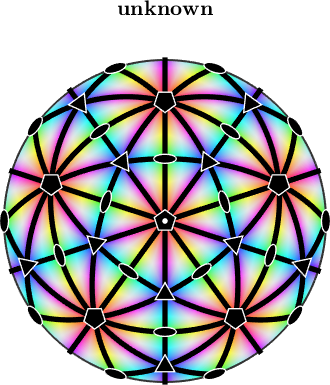Quasi symmetry edit page

By quasi crystals we mean materials with a non crystallographic symmetry group, e.g. including a pentagonal or decagonal symmetry axis. Such symmetry groups do not lead to perfect translational invariant lattices and hence are not crystals.

Setting up a non standard symmetry group can be done by specifying all its symmetry elements. To illustrate this definition lets consider the symmetry group corresponding to the dodecaeder which is generated by a 5 fold and a 3 fold symmetry axis.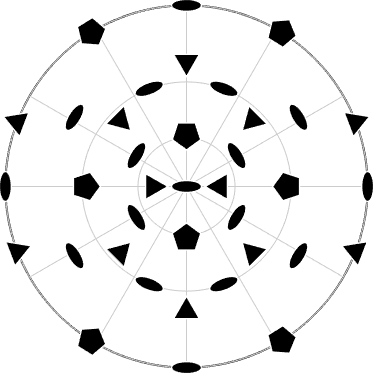Many of the MTEX methods do work also with quasi symmetries. E.g. we can identify the fundamental sector by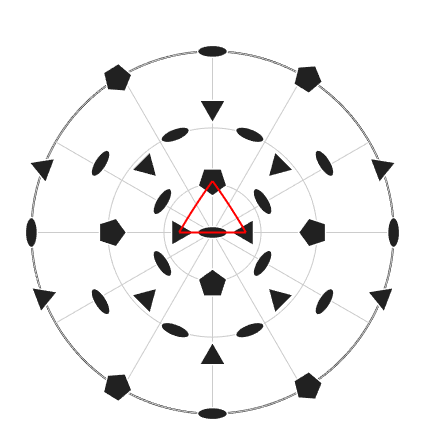the fundamental region is orientation space, which is exactly a dodecaeder,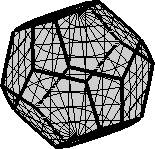or define an approbiate color key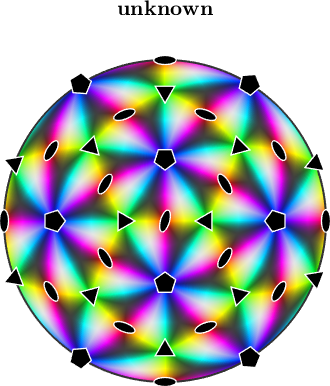We may also choose a different setup where the 5 fold axis is aligned parallel to z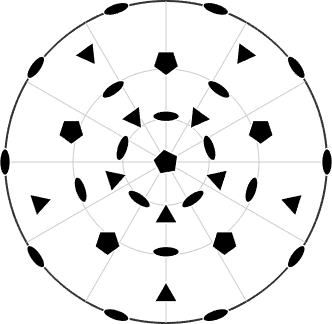or include the inversion